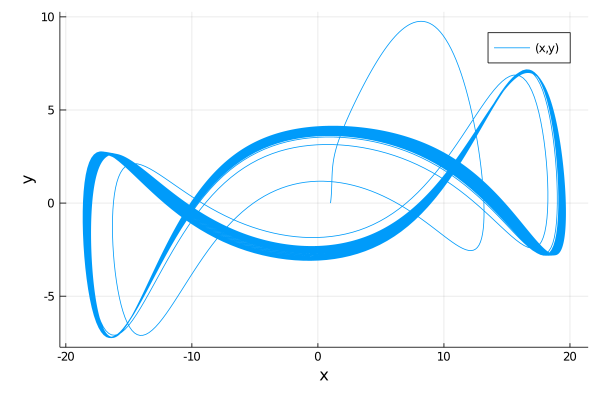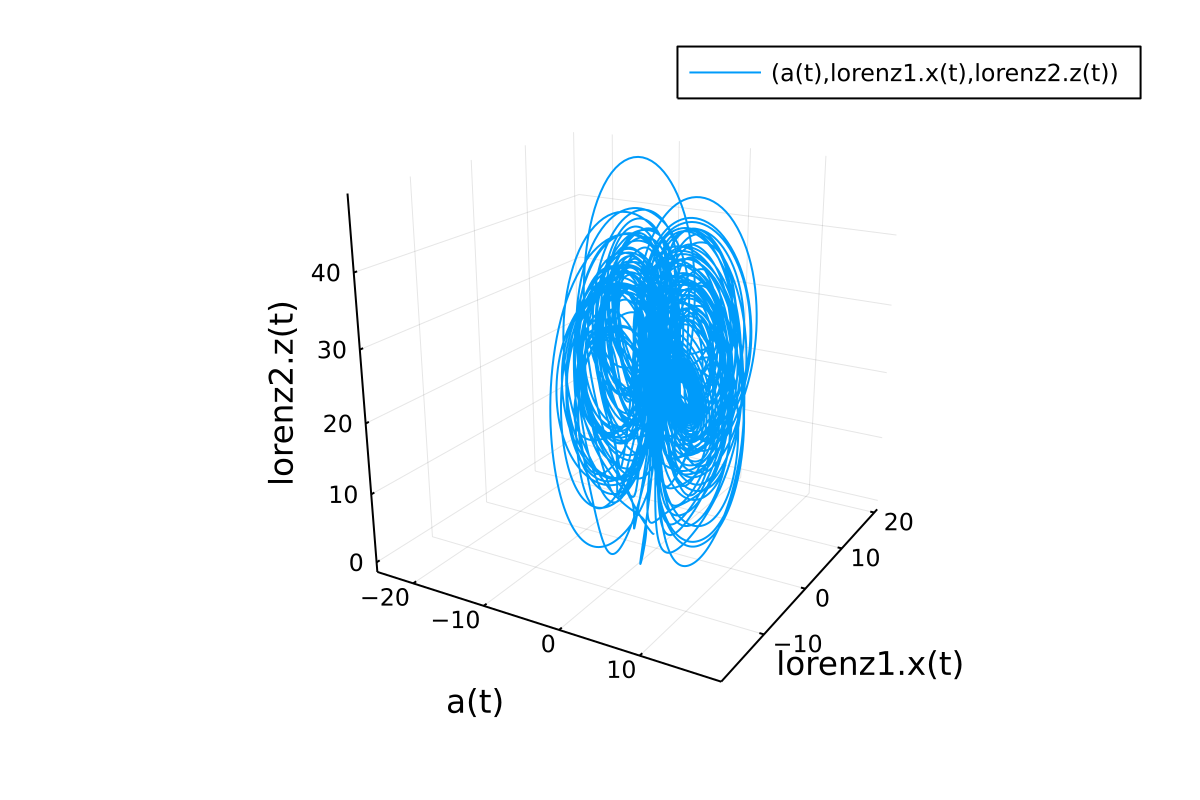# Modelingtoolkit.jl

An acausal modeling framework for automatically parallelized scientific machine learning (SciML) in Julia. A computer algebra system for integrated symbolics for physics-informed machine learning and automated transformations of differential equations
Alternatives To Modelingtoolkit.jl
Julia42,536
20 hours ago4,619mitJulia
The Julia Programming Language
Mlxtend4,404956019 days ago49May 27, 2022129otherPython
A library of extension and helper modules for Python's data analysis and machine learning libraries.
Hedgehog Lab2,323
3 months ago33apache-2.0TypeScript
Run, compile and execute JavaScript for Scientific Computing and Data Visualization TOTALLY TOTALLY TOTALLY in your BROWSER! An open source scientific computing environment for JavaScript TOTALLY in your browser, matrix operations with GPU acceleration, TeX support, data visualization and symbolic computation.
Modelingtoolkit.jl1,214
a day ago358otherJulia
An acausal modeling framework for automatically parallelized scientific machine learning (SciML) in Julia. A computer algebra system for integrated symbolics for physics-informed machine learning and automated transformations of differential equations
Paperai915
2 months ago10March 12, 2022apache-2.0Python
📄 🤖 Semantic search and workflows for medical/scientific papers
a year ago1
Armadillo: fast C++ library for linear algebra & scientific computing - http://arma.sourceforge.net
Workshopscipy396
5 years agomitJupyter Notebook
A workshop for scientific computing in Python. ( December 2017 )
Pdebench392
9 days ago6otherPython
PDEBench: An Extensive Benchmark for Scientific Machine Learning
18s096sciml268
a year ago1HTML
18.S096 - Applications of Scientific Machine Learning
Pycroscopy21612 months ago82April 01, 202249mitJupyter Notebook
Scientific analysis of nanoscale materials imaging data
Alternatives To Modelingtoolkit.jl
Select To Compare

Alternative Project Comparisons

# ModelingToolkit.jl

ModelingToolkit.jl is a modeling framework for high-performance symbolic-numeric computation in scientific computing and scientific machine learning. It allows for users to give a high-level description of a model for symbolic preprocessing to analyze and enhance the model. ModelingToolkit can automatically generate fast functions for model components like Jacobians and Hessians, along with automatically sparsifying and parallelizing the computations. Automatic transformations, such as index reduction, can be applied to the model to make it easier for numerical solvers to handle.

For information on using the package, see the stable documentation. Use the in-development documentation for the version of the documentation which contains the unreleased features.

## Standard Library

For a standard library of ModelingToolkit components and blocks, check out the ModelingToolkitStandardLibrary

## High-Level Examples

First, let's define a second order riff on the Lorenz equations, symbolically lower it to a first order system, symbolically generate the Jacobian function for the numerical integrator, and solve it.

using DifferentialEquations, ModelingToolkit

@parameters t σ ρ β
@variables x(t) y(t) z(t)
D = Differential(t)

eqs = [D(D(x)) ~ σ * (y - x),
D(y) ~ x * (ρ - z) - y,
D(z) ~ x * y - β * z]

@named sys = ODESystem(eqs)
sys = structural_simplify(sys)

u0 = [D(x) => 2.0,
x => 1.0,
y => 0.0,
z => 0.0]

p = [σ => 28.0,
ρ => 10.0,
β => 8 / 3]

tspan = (0.0, 100.0)
prob = ODEProblem(sys, u0, tspan, p, jac = true)
sol = solve(prob)
using Plots
plot(sol, idxs = (x, y))This automatically will have generated fast Jacobian functions, making it more optimized than directly building a function. In addition, we can then use ModelingToolkit to compose multiple ODE subsystems. Now, let's define two interacting Lorenz equations and simulate the resulting Differential-Algebraic Equation (DAE):

using DifferentialEquations, ModelingToolkit

@parameters t σ ρ β
@variables x(t) y(t) z(t)
D = Differential(t)

eqs = [D(x) ~ σ * (y - x),
D(y) ~ x * (ρ - z) - y,
D(z) ~ x * y - β * z]

@named lorenz1 = ODESystem(eqs)
@named lorenz2 = ODESystem(eqs)

@variables a(t)
@parameters γ
connections = [0 ~ lorenz1.x + lorenz2.y + a * γ]
@named connected = ODESystem(connections, t, [a], [γ], systems = [lorenz1, lorenz2])
sys = structural_simplify(connected)

u0 = [lorenz1.x => 1.0,
lorenz1.y => 0.0,
lorenz1.z => 0.0,
lorenz2.x => 0.0,
lorenz2.y => 1.0,
lorenz2.z => 0.0,
a => 2.0]

p = [lorenz1.σ => 10.0,
lorenz1.ρ => 28.0,
lorenz1.β => 8 / 3,
lorenz2.σ => 10.0,
lorenz2.ρ => 28.0,
lorenz2.β => 8 / 3,
γ => 2.0]

tspan = (0.0, 100.0)
prob = ODEProblem(sys, u0, tspan, p)
sol = solve(prob)

using Plots
plot(sol, idxs = (a, lorenz1.x, lorenz2.z))# Citation

@misc{ma2021modelingtoolkit,
title={ModelingToolkit: A Composable Graph Transformation System For Equation-Based Modeling},
author={Yingbo Ma and Shashi Gowda and Ranjan Anantharaman and Chris Laughman and Viral Shah and Chris Rackauckas},
year={2021},
eprint={2103.05244},
archivePrefix={arXiv},
primaryClass={cs.MS}
}

Popular Scientific Projects
Popular Machine Learning Projects
Popular Science Categories
Related Searches

Get A Weekly Email With Trending Projects For These Categories
No Spam. Unsubscribe easily at any time.
Machine Learning
Julia
Optimization
Scientific
Computer Algebra